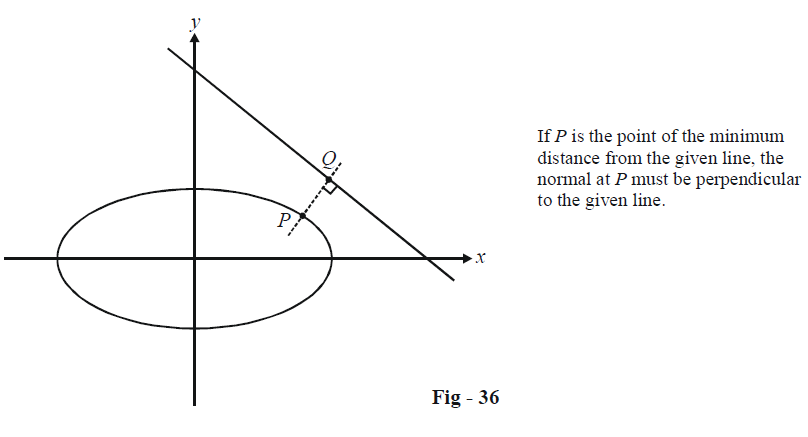# Examples On Normals To Ellipses Set-1

Go back to  'Ellipse'

Example - 32

What is the farthest distance at which a normal to the ellipse \begin{align}\frac{{{x^2}}}{{{a^2}}} + \frac{{{y^2}}}{{{b^2}}} = 1\end{align} can lie from the centre of the ellipse ?

Solution: Any normal to the ellipse is of the form

$ax\sec \theta - by\,{\rm{cosec }}\theta = {a^2} - {b^2}$

The distance of this normal from the centre (0, 0) is

$d = \frac{{\left| {{a^2} - {b^2}} \right|}}{{\sqrt {{a^2}{{\sec }^2}\theta + {b^2}\,{\rm{cose}}{{\rm{c}}^2}\,\theta } }}$

We need to find the maximum value of d, or equivalently, the minimum value of

$f(\theta ) = {a^2}{\sec ^2}\theta + {b^2}{\rm{cose}}{{\rm{c}}^2}\,\theta$

We have

\begin{align} & {f}'(\theta )=2{{a}^{2}}{{\sec }^{2}}\theta \tan \theta -2{{b}^{2}}\text{cose}{{\text{c}}^{2}}\theta \cot \theta \\ & \Rightarrow\quad {f}'(\theta )=0~when\;{{\tan }^{2}}\theta =\frac{b}{a} \\ &\qquad\qquad\qquad\quad \Rightarrow\quad \tan \theta =\pm \sqrt{\frac{b}{a}} \\ \end{align}

Verify that at this value of $$\theta ,f''(\theta )$$ is positive so that this $$\theta$$ indeed gives us the minimum value of $$f(\theta ).$$

Now,

\begin{align}&\qquad{f_{\min }}(\theta ) = {a^2}{\sec ^2}{\theta _{\min }} + {b^2}{\rm{cose}}{{\rm{c}}^2}\,{\theta _{\min }}\\&\qquad\quad\qquad{\rm{ }} = {a^2}(1 + {\tan ^2}{\theta _{\min }}) + {b^2}(1 + {\cot ^2}{\theta _{\min }})\\&\qquad\quad\qquad{\rm{ }} = {(a + b)^2}\\& \;\;\Rightarrow \quad {d_{\max }} = \frac{{\left| {{a^2} - {b^2}} \right|}}{{\sqrt {{f_{\min }}(\theta )} }}\\&\qquad\quad\qquad{\rm{ }} = \frac{{\left| {{a^2} - {b^2}} \right|}}{{\left( {a + b} \right)}}\\&\qquad\quad\qquad{\rm{ }} = \left| {a - b} \right|\end{align}

Example – 33

Find the point on the ellipse $$\frac{{{x^2}}}{6} + \frac{{{y^2}}}{3} = 1$$ whose distance from the line $$x + y = 7$$ is minimum.

Solution: Any point on the given ellipse can be assumed to be $$P(\sqrt 6 \cos \theta ,\,\sqrt 3 \sin \theta ).$$ From the following figure, observe that for the distance of P from the given line to be minimum, the normal at P must be perpendicular to the given line.The equation of the normal at P, using parametric form, is

$(\sqrt 6 \sec \theta )x - (\sqrt 3 {\rm{cosec}}\,\theta )y = 3$

whose slope is

${m_N} = \sqrt 2 \tan \theta$

If the normal is perpendicular to $$x + y = 7,$$ we have

\begin{align}&\quad\qquad{m_N} = 1\\ &\Rightarrow \quad \tan \theta = \frac{1}{{\sqrt 2 }}\\ &\Rightarrow \quad\sin \theta = \frac{1}{{\sqrt 3 }}\;and\;\cos \theta = \frac{{\sqrt 2 }}{{\sqrt 3 }}\end{align}

Thus, the point P is $$(a\cos \theta ,\,b\sin \theta ) \equiv (2,\,1).$$